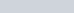• 77
•
• 1
•
•
•
•
•
78
Shares

# Conservative and non-conservative forces

In this article, we will discuss and think about potential energy and its definition, what are conservative and non-conservative forces? and we will learn about conservative field theory. so let’s start…Let’s choose a system, a system is basically a part of the universe under study, it can be a block, collection of blocks, it can be earth and sun taken as a system and we can imagine much more, Now we are analyzing all the forces that are acting on this system.  We can classify all the forces into external and internal forces.

Now we can classify internal forces into two types conservatives and non-conservative, let’s see what are these.

## Conservative forces

A force is defined to be conservative if the work done by the force is zero in a closed path and also work done by the force depends only upon initial and final positions, not on the path.

From the above points, we can find out that gravitational force, spring force, intermolecular force, electrostatic force on a charge, magnetic force on a charge are all conservative.

Also, every constant force (constant magnitude and direction) will be conservative as work done by it on a closed path will be zero.

# Non-conservative forces

Forces inside a system that aren’t conservative are non-conservative forces,

These forces cause energy mechanical energy (kinetic and potential energy) loss inside the system in form of heat energy, sound energy, etc.

Kinetic friction is the only non-conservative force observed in classical physics.

# Observations of conservative and non-conservative forces

Now there is a beauty about conservative forces, let’s take earth and a block as a system for study and we take a block and slowly take it upwards from ground to a height “h” we did some work done against gravitational force (conservative force) we invested our energy and now if leave the block the gravitational force will do work and take the block form “h” height to the ground.

Qualitatively, if conservative force is present then it f we do any work we will get back the work, the work by us will be stored as potential energy in the system,

On the other hand suppose a black is placed on the floor now if we move the block a distance “s” then the kinetic friction will oppose the motion of block also the work done by us will not be reverted to us as the block will remain to that position and doesn’t return back or kinetic fiction doesn’t give us any work instead a part of the work done by us will be lost due to kinetic friction in form of heat, sound, etc.

# Potential energy

We had discussed the above the ideal of potential energy and how if conservative forces are present then the work done by us will be stored in the system.

In any system potential energy is some stored energy that appears if conservative force is present, potential energy is defined according to conservative field theory.

## Conservative field theory and potential energy definition

Corresponding to every conservative force inside a system potential energy is separately defined for every conservative force. The potential energy definition isi.e. work done by conservative force equals the change in potential energy of the system, this is the way potential energy is defined.

It should be clear that if two or more conservative forces as gravitational and electrostatic force are within system then we say that work done by gravitational force is equals to change in gravitational potential energy of system similarly work done by electrostatic force equals change in electrostatic potential energy of system.

From the above definition, we can see that we define the change in potential energy as -ve of work done by conservative force, therefore only change in potential energy can be evaluated whereas the term absolute potential energy is not defined anywhere but we can assign potential energy at a position as zero and then write potential energy of other positions w.r.t that.